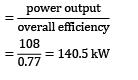Courses

# Test: Hydraulic Machines Level - 3

## 20 Questions MCQ Test Engineering Mechanics | Test: Hydraulic Machines Level - 3

Description
This mock test of Test: Hydraulic Machines Level - 3 for Civil Engineering (CE) helps you for every Civil Engineering (CE) entrance exam. This contains 20 Multiple Choice Questions for Civil Engineering (CE) Test: Hydraulic Machines Level - 3 (mcq) to study with solutions a complete question bank. The solved questions answers in this Test: Hydraulic Machines Level - 3 quiz give you a good mix of easy questions and tough questions. Civil Engineering (CE) students definitely take this Test: Hydraulic Machines Level - 3 exercise for a better result in the exam. You can find other Test: Hydraulic Machines Level - 3 extra questions, long questions & short questions for Civil Engineering (CE) on EduRev as well by searching above.
QUESTION: 1

### A Pelton wheel having semi-circular buckets functions under a head of 150 m and consumes 50 liters of water per second. If 60 cm diameter wheel turns 600 revolutions per minute, make calculations for the hydraulic efficiency of the wheel. Presume coefficient of velocity KV = unity.(A) 90(B) 91

Solution: Velocity of the jet,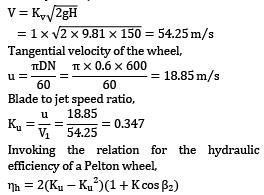Tangential velocity of the wheel,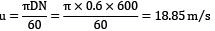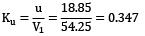Invoking the relation for the hydraulic efficiency of a Pelton wheel,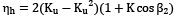Since the buckets are semi-circular, theoretically the jet gets deflected through 180° and therefore, the blade angle at exit β2 = 0°. Further, neglecting friction at the bucket surfaces

(K = 1)

ηh = 2 × (0.347 − 0.3472)(1 + cos 0°)

= 0.906 or 90.6%

Question_Type: 5

QUESTION: 2

### A Pelton wheel works at the foot of a dam because of which the head available at the nozzle is 220 m. The nozzle diameter is 20 cm and the coefficient of velocity is 0.98. The wheel operates at 250 rev/min and develops 3.75 MW of power. Assuming that the blade outlet angle is 16° and the jet speed ratio is 0.46, make calculations for the wheel diameter at the pitch circle of the blades, and the turbine efficiency.

Solution: The power output P and the torque T on the shaft of a Pelton wheel are given by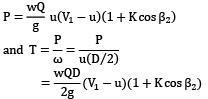It is seen from the above expressions that power output varies parabolically and the torque linearly with wheel speed u.

Nozzle outlet velocity,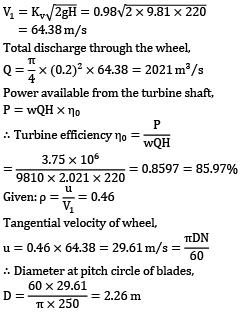Total discharge through the wheel,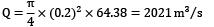Power available from the turbine shaft, P = wQH × η0

∴ Turbine efficiency η0 =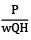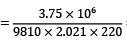= 0.8597 = 85.97%

Given: ρ = u / V1

= 0.46

Tangential velocity of wheel,

u = 0.46 × 64.38 = 29.61 m⁄s =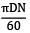∴ Diameter at pitch circle of blades,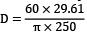= 2.26 m

QUESTION: 3

### A Pelton wheel is required to develop 4000 kW at 400 revs/min, operating under an available head of 350 m. There are two equal jets and the bucket deflection angle is 165°. Calculate the bucket pitch circle diameter, the cross-sectional areas of each jet. Make the following assumptions: (i) Overall efficiency is 85% when the water is discharged from the wheel in a direction parallel to the axis of rotation. (ii) Coefficient of velocity of the nozzle Kv = 0.97 and the blade speed ratio Ku = 0.46. (iii) Relative velocity of water at exit from the bucket is 0.86 times the relative velocity at inlet.

Solution: Power available from the turbine shaft

P = wQH × η0

⇒ 4000 × 103 = (9810 × Q × 350) × 0.85

∴ Total discharge though the wheel,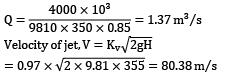The total discharge through the wheel must be equal to the discharge through the jets.

Thus, Q = nAV1 ; 1.37 = 2A × 80.38

∴ Area of each jet,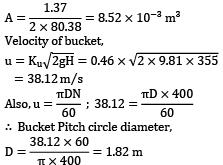Velocity of bucket,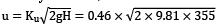= 38.12 m/s

Also,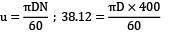∴ Bucket Pitch circle diameter,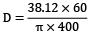= 1.82 m

QUESTION: 4

At a particular hydroelectric power plant, water available under a head of 250 m is delivered to the power house through three pipes each 2500 m long. Through these pipes, friction loss is estimated to be 20 m.

The project is required to produce a total shaft output of 13.25 MW by installing a number of single jet Pelton wheels whose specific speed is not to exceed 38.5. The other pertinent data is Wheel speed = 650 revolutions per minute Ratio of bucket to jet speed = 0.46 Overall efficiency of the wheel = 85% For nozzle Cd = 0.94 and Kv = 0.97.

Pipe friction coefficient f = 0.005 in the

Formula hf =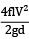Determine: (i) jet diameter (ii) Diameter of supply pipes

Solution: Net available head H = Hg − Hf = 250 − 20 = 230 m Invoking the relation for specific speed,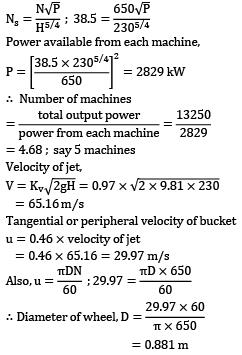Power available from each machine,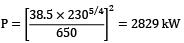∴ Number of machines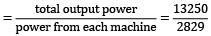= 4.68 ; say 5 machines

Velocity of jet,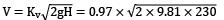= 65.16 m/s

Tangential or peripheral velocity of bucket u = 0.46 × velocity of jet = 0.46 × 65.16 = 29.97 m/s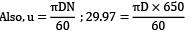∴ Diameter of wheel, D =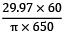= 0.881m

Power available from the turbine, per machine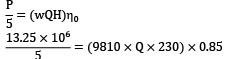∴ Discharge through each wheel,

Q =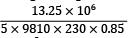= 1.38 m3⁄s

Also, Q = Cd √2gH × area of jet ;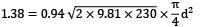Hence jet diameter, d = 0.167 m

Total discharge for 5 machines = 5 × 1.38 = 6.9 m3/s

Discharge per pipe =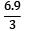= 2.3 m3/s

From the Darcy relation, loss of head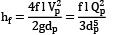where the subscript p refers to pipe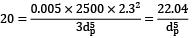∴ Diameter of pipe, dp =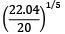=1.019 m

QUESTION: 5

An outward radial flow impulse turbine has nozzles with a total area of 10 cm2 . The guide vanes make an angle of 20° to the wheel tangent at exit. The inner and outer diameters are 0.5 m and 0.7 m respectively. The moving vanes have an outlet angle of 20° to the wheel tangent. The turbine develops 11.75 kW at the shaft running at 620 rpm under a head of 60 m, at a discharge of 0.03 m3⁄s. The water at discharge end leaves the runner in a forward direction and is inclined at 15° to the radius. Calculate

(i) the head lost in the nozzle

(ii) the head lost by the bearing friction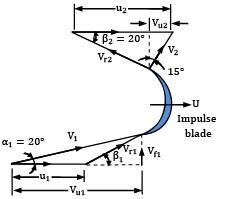Solution: Refer to Fig. in question for nomenclature and vector diagrams.

Analysis at inlet: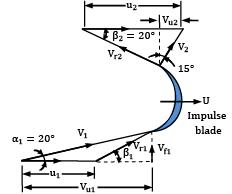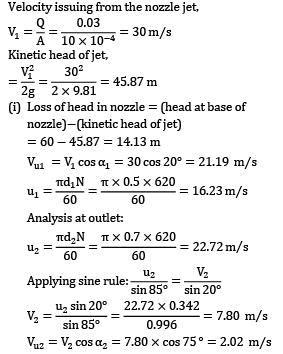Work done (or head utilized) per unit of weight of water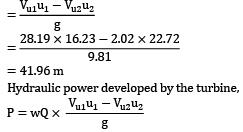= 9810 × 0.03 × 41.96 = 12350 W

= 12.35 kW

(ii) Power lost due to friction at the bearings = water power − brake power

= 12.35 − 11.75 = 0.60 kW If hf is the head loss in bearing,

then 0.60 × 103

= 9810 × 0.03 × hf ;

hf ≈ 2.04 m

QUESTION: 6

An inward flow reaction turbine works under a total head of 28 m. The velocity of wheel periphery at inlet is 15 m/s. The outlet pipe of the turbine is 30 cm in diameter and the turbine is supplied with 250 liters of water per second. The radial velocity of flow through the wheel is the same as velocity in outlet pipe. Neglecting friction, determine

a. vane angle at inlet

b. guide vane angle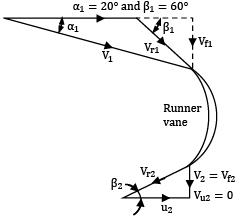Solution: Discharge = 250 litres per second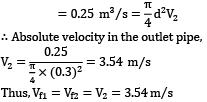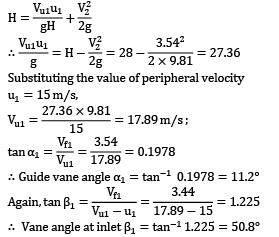QUESTION: 7

Two inward flow turbine runners have the same diameter 0.75 m and work under the same head with a velocity of flow of 6 m/s.

One runner operates at 450 rpm and has an inlet blade angle of 60-degree. Determine the speed (in rpm) at which the other turbine should run if its inlet blade angle is 105-degree. Assume that both the turbines have the same efficiency and radial discharge at outlet.

Solution: First runner: Velocity of vane tip at inlet,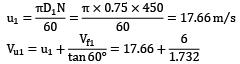= 21.12 m ⁄ s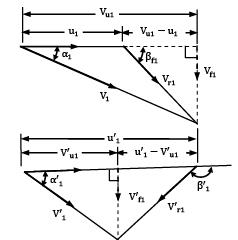As the discharge is radial at outlet Work done⁄kg

= Vu1u1 = 21.12 × 17.66

= 372.98 Nm

Second Runner: As both the turbines run under the same head and have the same efficiency, work done by the second runner will also be equal to 372.98 Nm. The flow velocity is also the same for both the runners. That is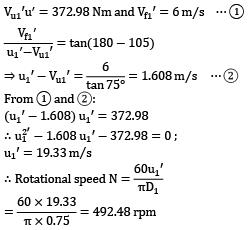QUESTION: 8

The runner of an inward flow reaction turbine is of 45 cm diameter and 5 cm width at the outer periphery; the corresponding dimensions at the inner periphery are 30 cm and 7.5 cm respectively, and vanes occupy 8% of the periphery. The guide vane angle is 25° to the tangent to the runner and the moving vanes have an inlet angle of 95° (vanes inclined forward to the direction of motion) and an exit angle of 30°. Hydraulic and mechanical friction losses respectively amount to 10% and 5% of the supply head, and the pressure in the outer casing is 55 cm more than that at discharge from the runner. You are required to work out the speed of the runner for no shocks at entry and the power available at the turbine shaft.

Refer to Fig. for nomenclature and the vector diagrams.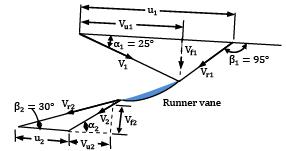Solution: From continuity considerations, (area at inlet) × Vf1 = (area at outlet) × Vf2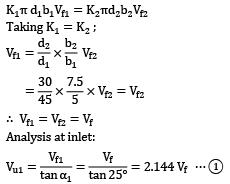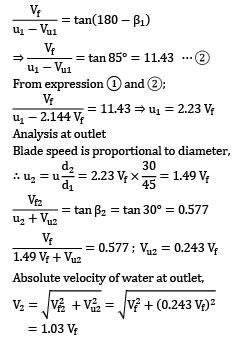From energy balance, Head supplied= work done or head utilized + energy at outlet (The head loss in the runner is being neglected)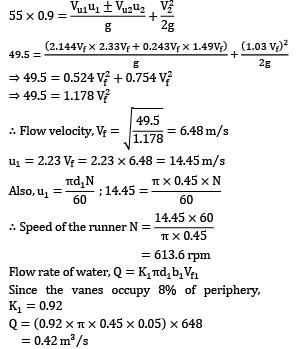Power developed by the turbine,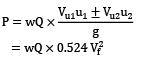= 9810 × 0.42 × 0.524 × (6.48)2

= 90657 W

Since the mechanical losses amount to 5% Brake power = 90657 × 0.95 = 86124 W ≈ 86 kW

QUESTION: 9

For a Kaplan turbine with runner diameter 4 m, the discharge is 60 m3⁄s and the hydraulic and mechanical efficiencies are stated to be 90 percent and 94 percent respectively. The diameter of the boss is 0.3 times the runner diameter and the speed ratio is 2.0. Assuming that discharge is free and there is no swirl at outlet, calculate the specific speed.

Solution: If Do is the outside diameter of the runner and Db is the diameter of hub or boss, then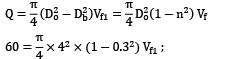Flow velocity Vf1 = 5.25 m/s Let H represent the net available head on the turbine.

Then In the absence of swirl at outlet,

V2 = Vf2 = 5.25 m⁄s Since hydraulic efficiency is 90%, ∴ 10% of the head leaves as kinetic head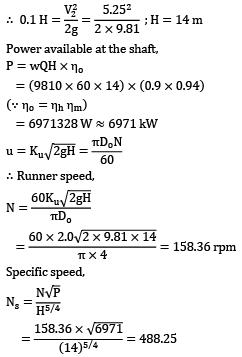QUESTION: 10

A propeller turbine has been designed to develop 22000 kW under a head of 20 m whilst turning 150 revolutions per minute. The relevant data is: hydraulic efficiency 95%; overall efficiency 88%; outer diameter 4.5 m and diameter of the hub 2 m. Determine the runner exit vane angles at the hub and at the outer periphery. Assume that the turbine discharges without whirl at exit.

Refer Fig. for nomenclature and velocity vector diagrams.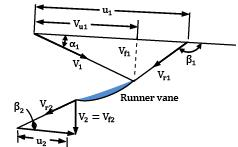Solution: Power available at the shaft, P = wQH × ηo 22000 × 103 = 9810 × Q × 20 × 0.88

∴ Flow rate Q = 127.42 m3⁄s

If Do is the outside diameter of the runner and Db is diameter of hub or boss, then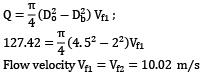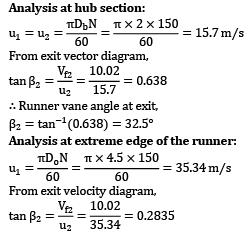Hence runner vane angle at exit, β2 = tan−1(0.2835) = 15.85°

QUESTION: 11

A Kaplan turbine develops 2250 kW under a net head of 5.5 m and with overall efficiency 87 percent. The draft tube has a diameter of 2.8 m at its inlet and has an efficiency of 78 percent. In order to avoid cavitation, the pressure head at entry to the draft tube must not drop more than 4.5 m below atmosphere. Calculate the maximum height at which the runner may be set above the tail race level.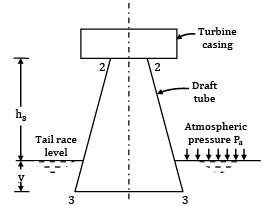Solution: Power available from the turbine shaft,

P = wQH × η 2250 × 103 = (9810 × Q × 5.5) × 0.87;

Q = 43.93 m3⁄s

Considering the equation,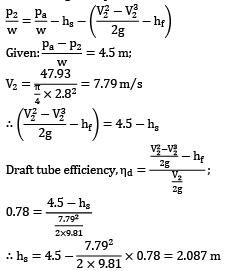QUESTION: 12

A turbine is to operate under a head of 25 meters at 200 rpm. The discharge is 9 m3/s. If the turbine efficiency is 90% determine: (i) specific speed of the turbine (ii) power developed under a head of 20 meters

Solution: Power available at the turbine shaft,

P = wQH × ηo = (9810 × 9 × 25) × 0.9

= 1986 × 103 W

Specific speed,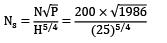= 159.44

Since the specific speed lies in the range 50-250, the turbine is a Francis turbine.

Since we are concerned with the same machine, we equate the relations for unit power.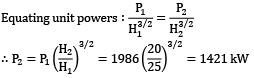QUESTION: 13

A hydro-electric power station required to generate 60,000 kW at 50 cycle frequency and at the available head of 20 m suggests the use of Kaplan turbine for which specific speed is 600 units. Make calculations for:

(i) hub diameter of the turbine assuming flow ratio 0.6 and that hub diameter of the wheel is 0.35 times the outside diameter

(ii) maximum safe elevation of runner to avoid cavitation taking vapour pressure 0.26 m of water and critical value of Thomas’s cavitation factor 0.7

Solution: Power at the shaft, P = wQH × ηo

∴ Discharge through the turbine,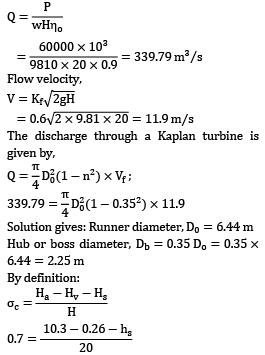⇒ hs = −3.96 m

The negative sign suggests that the turbine is to be installed below the tail race level. Kinetic energy head at draft tube inlet,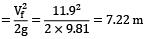Hence turbine should be placed (7.22 + 3.96) = 11.6 m below the tail race level.

QUESTION: 14

A water turbine is stated to have 50 percent degree of reaction. What is the physical meaning of this statement? Classify the Pelton, Francis and Kaplan runners according to the ranges of degree of reaction. Does the specific speed increase or decrease with an increase in the degree of reaction of a hydraulic turbine?

The velocity and pressure head at the entrance to the volute casing of a Francis turbine are 10 m/s and 240 m, and the elevation above tail race is 5 m. The shaft is vertical and the machine discharges 15 m3⁄s when turning 450 revolutions per minute. The mean diameter for the runner blades is 2 m and the width at inlet is 28 cm. If the discharge is to be axial, calculate:

(i) the specific speed

(ii) the degree of reaction

Assume values for the hydraulic and overall efficiencies are 94% and 92% respectively.

Solution: The statement that a water turbine has a 50 percent degree of reaction implies that the pressure drops in the rotor and guide blades are equal. The value of degree of reaction characterizes the design of a turbomachine. In a pure impulse turbine, there is no change of static pressure and degree of reaction R = 0. For a Francis runner: 0 < r="" />< 1.="" for="" a="" kaplan="" runner:="" 0.5="" />< r="" />< 1.="" the="" specific="" speed="" of="" a="" turbine="" increases="" with="" an="" increase="" in="" its="" degree="" of="" />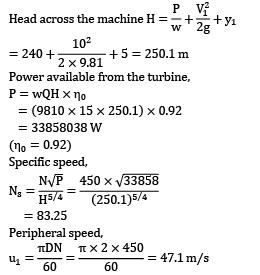Assuming axial discharge, hydraulic efficiency,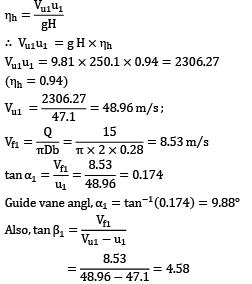∴ Rotor vane angle at inlet, β1 = tan−1 4.58 = 77.68° Degree of reaction,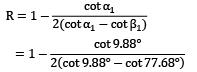= 0.48 or 48%

QUESTION: 15

Why pumps are generally less efficient than turbines?

A centrifugal pump is required to deliver 50 litres of water per second to a height of 30 m through a 100 m long pipe of 15 cm diameter. The inlet losses in the suction pipe are estimated to be 0.35 m. Assuming an overall efficiency of 70 percent and taking Darcy’s friction coefficient 0.015 for the pipeline, determine the power required to drive the pump, in kW.

Solution: Flow is of accelerating type in turbines and of decelerating type in pumps. As such, the tendency for boundary layer separation and eddy formation increases the hydraulic loss in pumps. Besides, turbines are usually of larger size than pumps and that result in reduced friction loss.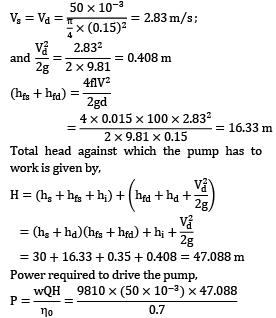= 32953 W ≈ 32.95 kW

QUESTION: 16

A centrifugal pump with impeller diameter 20 cm at outlet and width of outlet passage 2 cm has swept back blades at 25° to the tangent to the periphery. Calculate the power (in Watt) requirement while operating at 300 rpm under the condition of maximum efficiency.

Solution: The energy transfer for a centrifugal pump is given by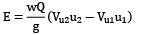(where u2 is the blade peripheral speed at outlet, Vf2 is the outlet velocity of flow and β2 is the blade angle at outlet measured with respect to the tangent) For an axial or radial fluid entry (fluid entering the impeller with no whirl component), term Vu1u1 vanishes and the above expression takes the form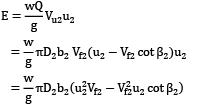The pump will be operating most efficiently if the energy transfer is maximum. The condition for maximum energy transfer can be worked out by differentiating (u22Vf2 − Vf22 u2 cot β2 ) with respect to Vf2 and setting the derivative to zero. That is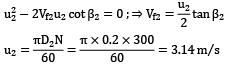Vf2 (for optimum pump performance)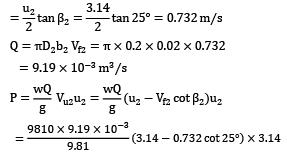⇒ P = 45.33 Nm ⁄ s = 45.33W

QUESTION: 17

A single stage centrifugal pump discharge 0.45 m3 of water per minute producing a head of 15 m. A motor drives the pump and the tachometer registers a speed of 1250 rpm. At this speed the brake power necessary to drive the pump is 6 kW. If the number of revolutions is increased to 1450 rpm, find the new discharge, head and brake power.

Solution: Invoking non-dimensional coefficients for one particular machine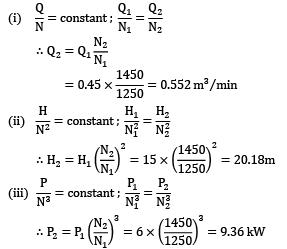QUESTION: 18

A single stage centrifugal pump runs at 500 rpm and delivers 300 m3⁄min of water against a head of 120 m. The pump impeller is 2 m in diameter and it has a positive suction lift (including the velocity head and friction) of 3 m. Laboratory tests are to be conducted on a model with 45 cm impeller and on a reduced head of 95 m. Calculate the speed, discharge and suction lift for the laboratory tests. Assume vapour head Hv = 0.34 m of water and atmospheric head Ha = 10.15 m of water.

Solution: Let suffix p and m respectively represent the prototype and model pump.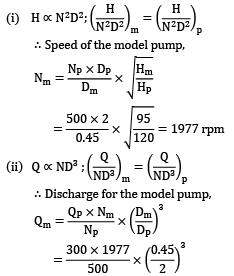= 13.51 m3⁄min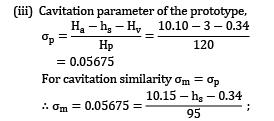hs = 4.418 m Hence the model should be tested with a positive suction lift of 4.418 m.

QUESTION: 19

A 4-stage centrifugal pump supplying water is to be designed for a total lift of 120 m when running at 1450 rpm; its discharge under these conditions is 0.24 m3 /s. The vanes are set back at an angle of 30° with the tangent to the wheel at outlet, and the impeller is surrounded by guide vanes. The water enters the vane passages in a radial direction, the velocity of flow through the impeller is 0.3 of the outlet peripheral velocity and the losses in the pump amount to one-third of the velocity head at discharge from the impeller. Find the width of the impeller at outlet, and the manometric efficiency.

Solution: Refer Fig. for nomenclature and velocity vector diagrams.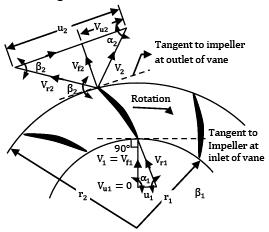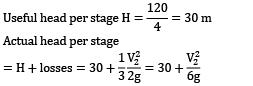The actual head equals the energy transfer per unit weight; referred to as Euler head. That is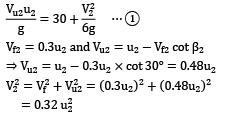Substituting values of V2 and Vu2 in expression ①, we get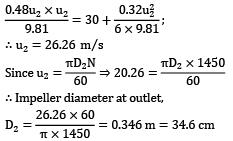Now, discharge Q

= πD2b2 × Vf2 ; 0.24

= π × 0.346 × b2 × (0.3 × 26.26)

∴ Width of impeller at outlet,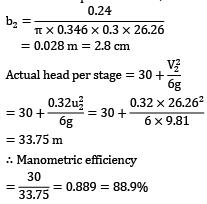QUESTION: 20

A multistage boiler feed pump is required to pump 115 × 103 kg of water per hour against a pressure difference of 3150 kN/m2 when running at a speed of 3000 rev/min. The density of preheated feed water is 950 kg/m3. If all the impellers are identical and the specific speed per stage is not to be less than 22, work out a. the diameter of the impeller b. the shaft power required to drive the pump.

It may be presumed that overall efficiency is 77% and peripheral velocity of the impeller is given by u = 0.96√2gh where h is the head per stage.

Solution: Volumetric flow rate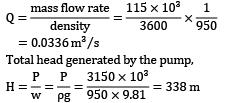(a) Let x be the number of stages. Then head created per stage, h = 338 / x Invoking the relation for specific speed,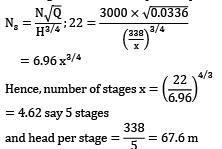(b) Peripheral or tip speed of the impeller,

u = 0.96√2gh

= 0.96 × √2 × 9.81 × 67.6

= 34.96 m⁄s

∴ Diameter of impeller,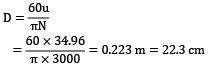(c) Power output of pump,

P = wQH

= (ρg)QH

= (950 × 9.81) × 0.0336 × 338

= 108150 W ≈ 108 kW

∴ Shaft power required to drive the pump,